International
Tables for
Crystallography
Volume A
Space-group symmetry
Edited by M. I. Aroyo

International Tables for Crystallography (2015). Vol. A, ch. 1.4, pp. 44-45

## Section 1.4.1.4.2. General aspects

H. Wondratscheke

#### 1.4.1.4.2. General aspects

| top | pdf |

The Hermann–Mauguin symbol for a space group consists of a sequence of letters and numbers, here called the constituents of the HM symbol. The first constituent is always a symbol for the conventional cell of the translation lattice of the space group (cf. Section 1.3.2.1for the definition of the translation lattice); the following constituents, namely rotations, screw rotations, rotoinversions, reflections and glide reflections, are marked by conventional symbols, cf. Table 2.1.2.1.1 Together with the generating translations of the lattice, the set of these symmetry operations forms a set of generating symmetry operations of the space group. The space group can thus be generated from its HM symbol.

The symmetry operations of the constituents are referred to the lattice basis that is used conventionally for the crystal system of the space group. The kind of symmetry operation can be read from its symbol; the orientation of its geometric element, cf. de Wolff et al. (1989, 1992), i.e. its invariant axis or plane normal, can be concluded from the position of the corresponding constituent in the HM symbol, as the examples in the following sections will show. The origin is not specified. It is chosen by the user, who selects it in such a way that the matrices of the symmetry operations appear in the most convenient form. This is often, but not necessarily, the conventional origin chosen in the space-group tables of this volume. The choice of a different origin may make other tasks, e.g. the derivation of the space group from its generators, particularly easy and transparent.

The first constituent (the lattice symbol) characterizes the lattice of the space group referred to the conventional coordinate system. (Each lattice can be referred to a lattice basis, also called a primitive basis: the lattice vectors have only integer coefficients and the lattice is called a primitive lattice.) Lattice vectors with non-integer coefficients can occur if the lattice is referred to a non-primitive basis. In this way similarities and relations between different space-group types are emphasized.

The lattice symbol of a primitive basis consists of an upper-case letter P (primitive). Lattices with conventional non-primitive bases are called centred lattices, cf. Section 1.3.2.4and Table 2.1.1.2. For these other letters are used: if the ab plane of the unit cell is centred with a lattice vector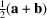, the letter is C; for ca centring [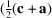as additional centring vector] the letter is B, and A is the letter for centring the bc plane of the unit cell by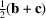. The letter is F for centring all side faces of the cell with centring vectors,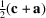and. It is I (German: innenzentriert) for body centring by the vector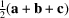and R for the rhombohedral centring of the hexagonal cell by the vectorsand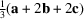. In 1985, the letter S was introduced as a setting-independent centring symbol' for monoclinic and orthorhombic Bravais lattices (cf. de Woff et al., 1985).

To describe the structure of the HM symbols the introduction of the term symmetry direction is useful.

#### Definition

A direction is called a symmetry direction of a crystal structure if it is parallel to an axis of rotation, screw rotation or rotoinversion or if it is parallel to the normal of a reflection or glide-reflection plane. A symmetry direction is thus the direction of the geometric element of a symmetry operation when the normal of a symmetry plane is used for the description of its orientation.

The corresponding symmetry operations [the element set of de Wolff et al. (1989& 1992)] specify the type of the symmetry direction. The symmetry direction is always a lattice direction of the space group; the shortest lattice vector in the symmetry direction will be called q.

If q represents both a rotation or screw rotation and a reflection or glide reflection, then their symbols are connected in the HM symbol by a slash /', e.g. 2/m or 41/a etc.

The symmetry directions of a space group form sets of equivalent symmetry directions under the symmetry of the space group. For example, in a cubic space group the a, b and c axes are equivalent and form the set of six directions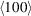: ,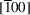,  etc. Another set of equivalent directions is formed by the eight space diagonals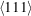: ,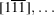. If there are twofold rotations around the twelve face diagonals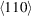, as in the space group of the crystal structure of NaCl,forms a third set of 12 symmetry directions.2

Instead of listing the symmetry operations (element set) for each symmetry direction of a set of symmetry directions, it is sufficient to choose one representative direction of the set. In the HM symbol, generators for the element set of each representative direction are listed.

It can be shown that there are zero (triclinic space groups), one (monoclinic), up to two (trigonal and rhombohedral) or up to three (most other space groups) sets of symmetry directions in each space group and thus zero, one, two or three representative symmetry directions.

The non-translation generators of a symmetry direction may include only one kind of symmetry operation, e.g. for twofold rotations 2 in space group P121, but they may also include several symmetry operations, e.g. 2, 21, m and a in space group C12/m1. To search for such directions it is helpful simply to look at the space-group diagrams to find out whether more than one kind of symmetry operation belongs to the generators of a symmetry direction. In general, only the simplest symbols are listed (simplest-operation rule): if we use >' to mean has priority', then pure rotations > screw rotations; pure rotations > rotoinversions; reflection.3 The space group mentioned above is conventionally called C12/m1 and not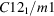or C12/a1 or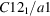.

The position of a plane is fixed by one parameter if its orientation is known. On the other hand, fixing an axis of known direction needs two parameters. Glide components also show two-dimensional variability, whereas there is only one parameter of a screw component. Therefore, reflections and glide reflections can better express the geometric relations between the symmetry operations than can rotations and screw rotations; reflections and glide reflections are more important for HM symbols than are rotations and screw rotations. The latter are frequently omitted to form short HM symbols from the full ones.

The second part of the full HM symbol of a space group consists of one position for each of up to three representative symmetry directions. To each position belong the generating symmetry operations of their representative symmetry direction. The position is thus occupied either by a rotation, screw rotation or rotoinversion and/or by a reflection or glide reflection.

The representative symmetry directions are different in the different crystal systems. For example, the directions of the basis vectors a, b and c are symmetry independent in orthorhombic crystals and are thus all representative, whereas a and b are symmetry equivalent and thus dependent in tetragonal crystals. All three directions are symmetry equivalent in cubic crystals; they belong to the same set and are represented by one of the directions. Therefore, the symmetry directions and their sequence in the HM symbols depend on the crystal system to which the crystal and thus its space group belongs.

Table 1.4.1.1gives the positions of the representative lattice-symmetry directions in the HM symbols for the different crystal systems.

 Table 1.4.1.1| top | pdf | The structure of the Hermann–Mauguin symbols for the space groups
 The positions of the representative symmetry directions for the different crystal systems are given. The description of the non-translational part of the HM symbol is always preceded by the lattice symbol, which in conventional settings is P, A, B, C, F, I or R. For monoclinic b setting and monoclinic c setting, cf. Section 1.4.1.4.4; the primitive hexagonal lattice is called H in this table.
Crystal system First positionSecond positionThird position
Triclinic (anorthic)   1 orMonoclinic b setting 1 b 1
Monoclinic c setting 1 1 c
Orthorhombic a b c
Tetragonal c a ab
Trigonal H lattice c a 1
or
c 1 ab
Trigonal, R lattice, hexagonal coordinates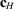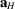or
Trigonal, R lattice, rhombohedral coordinates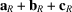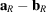Hexagonal c a ab
Cubic c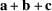ab

Examples of full HM symbols are (from triclinic to cubic), P12/c1, A112/m, F2/d2/d2/d,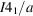,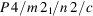,, P3m1,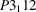,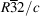,,and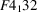.

There are crystal systems, for example tetragonal, for which the high-symmetry space groups display symmetry in all symmetry directions whereas lower-symmetry space groups display symmetry in only some of them. In such cases, the symmetry of the empty' symmetry direction is denoted by the constituent 1 or it is simply omitted. For example, instead of three symmetry directions in P4mm, there is only one in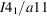, for which the HM symbol is usually written. However, in some trigonal space groups the designation of a symmetry direction by 1' () is necessary to maintain the uniqueness of the HM symbols.4

The HM symbols can not only describe the space groups in their conventional settings but they can also indicate the setting of the space group relative to the conventional coordinate system mentioned in Section 1.4.1.3.1. For example, the orthorhombic space group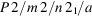may appear as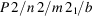or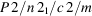or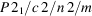or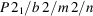or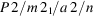depending on its orientation relative to the conventional coordinate basis. On the one hand this is an advantage, because the HM symbols include some indication of the orientation of the space group and form a more powerful tool than being just a space-group nomenclature. On the other hand, it is sometimes not easy to recognize the space-group type that is described by an unconventional HM symbol. In Section 1.4.1.4.5an example is provided which deals with this problem.

The full HM symbols describe the symmetry of a space group in a transparent way, but they are redundant. They can be shortened to the short HM symbols such that the set of generators is reduced to a necessary set. Examples will be displayed for the different crystal systems. The conventional short HM symbols still provide a unique description and enable the generation of the space group. For the monoclinic space groups with their many conventional settings they are not variable and are taken as standard for their space-group types. Monoclinic short HM symbols may look quite different from the full HM symbol, e.g. Cc instead of A1n1 or I1a1 or B11n or I11b.

The extended HM symbols display the additional symmetry that is often generated by lattice centrings. The full HM symbol denotes only the simplest symmetry operations for each symmetry direction, by the `simplest symmetry operation' rule; the other operations can be found in the extended symbols, which are treated in detail in Section 1.5.4and are listed in Tables 1.5.4.3(plane groups) and 1.5.4.4(space groups).

From the HM symbol of the space group, the full or short HM symbol for a crystal class of a space group is obtained easily: one omits the lattice symbol, cancels all screw components such that only the symbol for the rotation is left and replaces any letter for a glide reflection by the letter m for a reflection. Examples are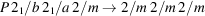and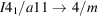.

If one is not yet familiar with the HM symbols, it is recommended to start with the orthorhombic space groups in Section 1.4.1.4.5. In the orthorhombic crystal system all crystal classes have the same number of symmetry directions and the HM symbols are particularly transparent. Therefore, the ortho­rhombic HM symbols are explained in more detail than those of the other crystal systems.

The following discussion treats mainly the HM symbols of space groups in conventional settings; for non-conventional descriptions of space groups the reader is referred to Chapter 1.5.

### References

Wolff, P. M. de, Belov, N. V., Bertaut, E. F., Buerger, M. J., Donnay, J. D. H., Fischer, W., Hahn, Th., Koptsik, V. A., Mackay, A. L., Wondratschek, H., Wilson, A. J. C. & Abrahams, S. C. (1985). Nomenclature for crystal families, Bravais-lattice types and arithmetic classes. Report of the International Union of Crystallography Ad-Hoc Committee on the Nomenclature of Symmetry. Acta Cryst. A41, 278–280.
Wolff, P. M. de, Billiet, Y., Donnay, J. D. H., Fischer, W., Galiulin, R. B., Glazer, A. M., Hahn, Th., Senechal, M., Shoemaker, D. P., Wondratschek, H., Wilson, A. J. C. & Abrahams, S. C. (1992). Symbols for symmetry elements and symmetry operations. Final report of the International Union of Crystallography Ad-Hoc Committee on the Nomenclature of Symmetry. Acta Cryst. A48, 727–732.
Wolff, P. M. de, Billiet, Y., Donnay, J. D. H., Fischer, W., Galiulin, R. B., Glazer, A. M., Senechal, M., Shoemaker, D. P., Wondratschek, H., Hahn, Th., Wilson, A. J. C. & Abrahams, S. C. (1989). Definition of symmetry elements in space groups and point groups. Report of the International Union of Crystallography Ad-Hoc Committee on the Nomenclature of Symmetry. Acta Cryst. A45, 494–499.